Select Page

# Timestamp Ordering With Examples

As we already That there are two rules for timestamp ordering,

Rule No. 01 is used when any transaction wants to perform Read(A) operation

• If WTS(A) > TS (Ti), then Ti Rollback
• Else (otherwise) execute R(A) operation and SET RTS (A) = MAX {RTS(A), TS(Ti)}

Rules No.2 is rules is used when a transaction needs to perform WRITE (A)

• If RTS(A) > TS (Ti), then Ti Rollback
• If WTS(A) > TS (Ti), then Ti Rollback
• Else (otherwise) execute W(A) operation and SET WTS (A) = TS(Ti)

Where “A” is some data

## Example of Timestamp ordering Protocol

Let Explain with Example, Look at following table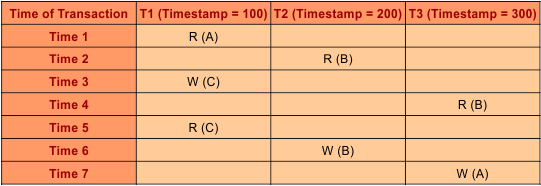### Solution:

Draw the following table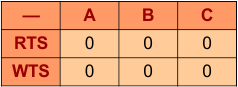In above table A,B,C are data values. And  Read and Write timestamp values are given “0”. As in the example table, time0 to time7 are given, let discuss one by one all.

At time 1,  the transaction 1 wants to perform read operation on data “A” then according to Rule No 01,

• WTS(A) > TS(T1) = 0>100 // condition false
• Go to else part and SET RTS (A) = MAX {RTS(A), TS(T1)} So,
• RTS (A) = MAX{0,100}= 100.
• So, finally RTS(A) is updated with 100

Updated table will be appear as following,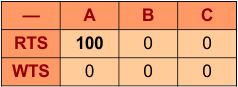At time 2,  the transaction 2 wants to perform read operation on data “B” then according to Rule No 01,

• WTS(B) > TS(T2) = 0>200 // condition false
• Go to else part and SET RTS (B) = MAX {RTS(B), TS(T2)} So,
• RTS (B) = MAX{0,200} = 200.
• So, finally RTS(B) is updated with 200

Updated table will be appear as following,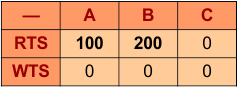At time 3,  the transaction 1 wants to perform write operation on data “C” then according to Rule No 02,

• RTS(C) > TS(T1) = 0>100 // condition false
• Go to second condition, WTS(C) > TS(T1) = 0>100 // again condition false
• Go to else part and SET WTS (C) = TS(T1) So,
• WTS (C) = TS(T1) = 100.
• So, finally WTS(C) is updated with 100

Updated table will be appear as following,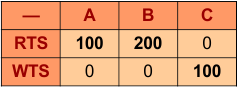At time 4,  the transaction 3 wants to perform read operation on data “B” then according to Rule No 01,

• WTS(B) > TS(T3) = 0>300 // condition false
• Go to else part and SET RTS (B) = MAX {RTS(B), TS(T3)} So,
• RTS (B) = MAX{200,300} = 300.
• So, finally RTS(B) replace 200 and updated with 300.

Updated table will be appear as following,At time 5,  the transaction T1 wants to perform read operation on data “C” then according to Rule No 01,

• WTS(C) > TS(T1) = 100>100 // condition false
• Go to else part and SET RTS (C) = MAX {RTS(C), TS(T1)} So,
• RTS (A) = MAX{0,100}= 100.
• So, finally RTS(C) is updated with 100

Updated table will be appear as following,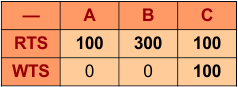At time 6,  the transaction 2 wants to perform write operation on data “B” then according to Rule No 02,

• RTS(B) > TS(T2) = 300>200 // condition True

According to Rule 2: if condition true then Rollback T2.

When T2 rollback then it never be resume, it will restart with new timestamp value. Keep in mind  T2 restart after completion of all running transactions, so in this example T2 will restart after completion of T3.

It happens due to confilict where the older transaction (T2) want to perform write operation on data “B” but  younger transaction (T3) already Read the same data “B”

Table will remain the sameAt time 7,  the transaction 3 wants to perform write operation on data “A” then according to Rule No 02,

• RTS(A) > TS(T3) = 100>300 // condition false
• Go to second condition, WTS(A) > TS(T3) = 100>300 // again condition false
• Go to else part and SET WTS (A) = TS(T3) So,
• WTS (A) = 300.
• So, finally WTS(A) is updated with 300

Updated table will be appear as following,Help Other’s By Sharing…